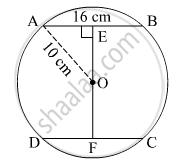Advertisement Remove all ads

# Radius of Circle is 10 Cm. There Are Two Chords of Length 16 Cm Each. What Will Be the Distance of These Chords from the Centre of the Circle ? - Geometry

Sum

Radius of circle is 10 cm. There are two chords of length 16 cm each. What will be the distance of these chords from the centre of the circle ?

Advertisement Remove all ads

#### SolutionLet the two chords be AB and CD.

AB = CD = 16 cm
Radius = AO = 10 cm
Let OE be the perpendicular drawn from the centre of the circle to the chord AB.
Perpendicular drawn from the centre of the circle to the chord bisects the chord.
So, AE = EB = 8 cm
In Δ AEO,

EO2 + AE2 = AO2

⇒ EO2 + 82 = 102

⇒ EO2 + 64 = 100

⇒ EO2 = 36

⇒ EO= 6 cm

we know that congruent chords of a circle are equidistant from the centre.
So, EO = OF = 6 cm
Distance of these chords from the centre is 6 + 6 = 12 cm.

Is there an error in this question or solution?
Advertisement Remove all ads

#### APPEARS IN

Balbharati Mathematics 2 Geometry 9th Standard Maharashtra State Board
Chapter 6 Circle
Practice Set 6.2 | Q 1 | Page 82
Advertisement Remove all ads
Advertisement Remove all ads
Share
Notifications

View all notifications

Forgot password?